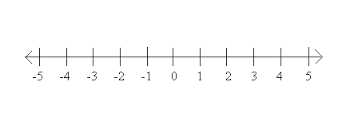top of page
Search

# ALGEBRA AND THE NUMBER LINE

## Don't Get This Wrong!In a number line, the numbers on the right of zero are positive while the numbers on the left of zeros are negative.As you move to the right, the number increases. On the other hand, if you move to the left, the number decreases.

Let’s compare between 1 and 2. Which number is bigger?Obviously, the answer is 2.

Now, let’s compare between -1 and -2. Which number is bigger?The answer is -1. Why?From -1 to -2, the number moves to the left on the number line and hence, it decreases. The number becomes more negative and hence, smaller.Addition and Subtraction Once you have understood this concept, you need to learn how to do addition and subtraction which involves negative numbers.What is 5 – 8?Let’s illustrate on a number line below.We shall start from 5. Minus 8 means we move 8 spaces to the left. So the number will end at -3. You can imagine -3 as shortage of 3. If you have 5 balls and you need to give 8 balls away. You will have shortage of 3 balls.Let’s look at another one.What is -6 + 8?We start from -6 first. Plus 8 means we move 8 spaces to the right. So we will end at positive 2. However, we do not write positive 2 as +2. We simply write as 2 which is positive by default.In fact, you may have realised that -6 + 8 is the same as 8 – 6. Yes, you can actually move the numbers around. But remember to bring the sign along! In this case, the minus sign belongs to 6. So when you move 6 to the back, the minus sign need to follow it. This explains how your child can do addition and subtraction involving negative numbers